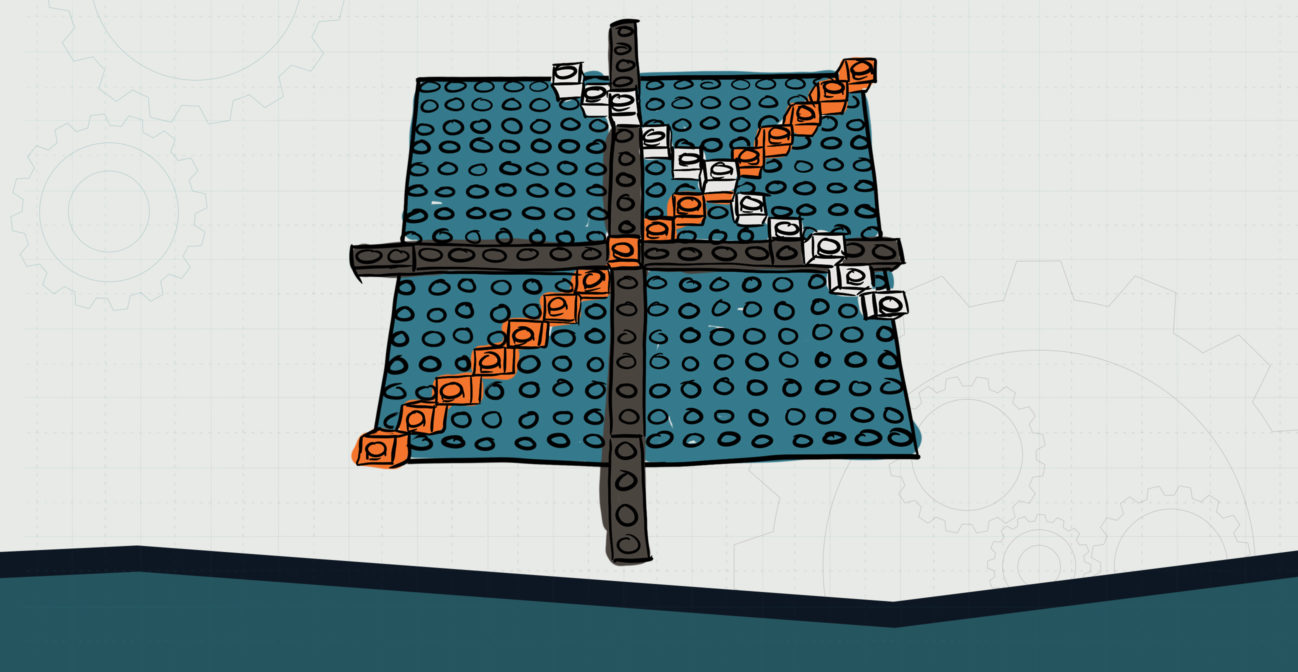# When Bricks Collide

## Bricks are used to investigate system of equations.Objective:
Child will be able to investigate system of equations to find their solutions using SOHO Brick graphs.

Essential Question:
How might we identify solutions to a set of equations using graphs?

Special Materials:
None

Bricks Required:

1x bricks of various sizes; 2 brick plates

Project Structure

Engage/Explain:

1. Have child graph a set of equations using SOHO Bricks.
2. Ask child a series of questions about the graphs and review:
1. What is the y-intercept of each graph?
2. What are the slopes of each graph?
3. Do the graphs intersect at all? Where? How do you know they intersect?
4. What does it mean to have two lines intersect?
3. Have child clearly identify and label the coordinates of intersection.

Explore:

1. Have child solve the system of equations they graphed, using algebra:
1. This can be done via a method of their choosing and should be supported and scaffolded.
2. Have child compare their solution from their equation to the intercept.
1. Ask child to explain what they see and what it means for the graph: mainly, that the solution to the equations is always the point of intersection between the two lines.

Explain/Elaborate:

1. Provide child with systems that do not have points of intersection, such as parallel lines.
2. Have child graph and see they do not intersect. Ask them the questions from the “Engage” section above.
3. Ask child to use observation to develop a way to determine if lines will have a solution or a point of intersection